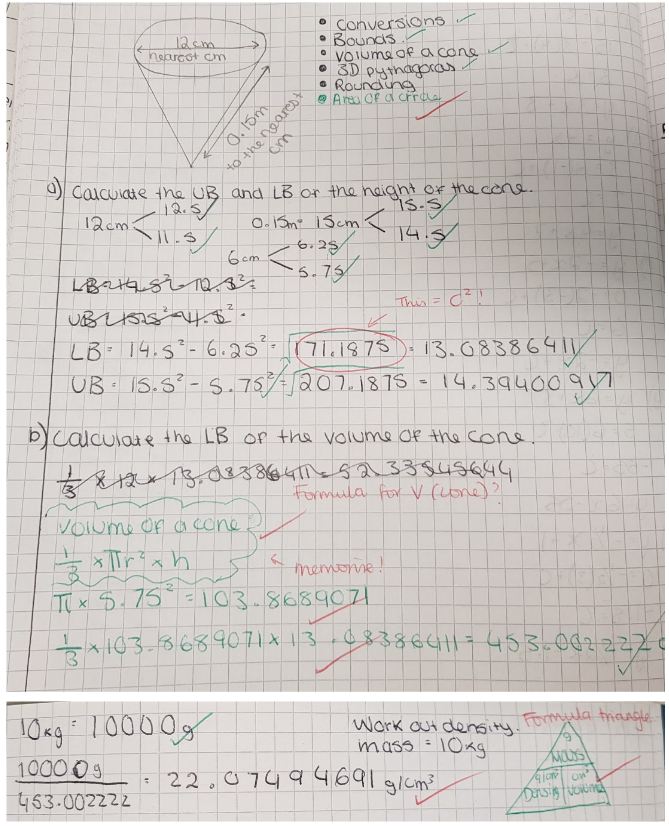top of page
Search

# Visualising Mathematics Sequencing: accounts of how we improved our Mathematics curriculum (part 3)

Zarah Kanwel

Deputy More Able Student Lead, Forest Gate Community School## Visualising the Mathematics Sequencing

The diagrams below visualise how the maths department has approached the sequencing of our long term plan (LTP). The aim is to ‘Carefully identify components of learning and sequence these across different years, which leads to students being able to access composite knowledge later on in their shared journey.’*

The question below is quite loaded and is a typical question posed to students in Year 9. In order to accurately answer this question, students must demonstrate a secure understanding on a number of prior ‘knowledge components’.It is very important therefore that teachers ensure the following:

1. Teach the previously linked knowledge components thoroughly and ensure students demonstrate a secured understanding

2. Revisit these knowledge components frequently through lesson starters and interim tests

The diagram below shows the links to other knowledge components belonging to various yearsThe list below shows some key considerations when approaching the question:

1. Students are given time to list topics they think they may need to answer this question

2. The shape had been rotated; as a lot of students seem to get confused if the shape is in a different orientation to what they are used to

3. Students require the radius for their calculation; diameter had been given instead so they can use their knowledge of diameters and radii from year 7 and 8 to calculate the radius

4. Students require the perpendicular height of the cone for their calculation; I have given them the slanted height so that they can use their learning of Pythagoras’ theorem from last year and Pythagoras’ Theorem in 3D from KO3 earlier this year to find the perpendicular height

5. Students require all measurements to be in the same units; I have given one measurement in cm whilst the other measurement is in m so they will need to be able to recognise this and convert one of the units, using their knowledge of converting units from year 7

6. The measurements of the cone may have been rounded by the person measuring; I have added “to the nearest cm” to each of the measurements so that students can use their knowledge of upper and lower bounds from KO1 earlier this year to take all possible measurements into account when carrying out calculations for the volume

7. Students need to know the formula for the volume of a cone as this was not given in the exam under the new syllabus; students were set flipped homework to learn the formula and were tested during the starter of the lesson

8. Once the upper and lower bounds of the volume are calculated, students must give only 1 final answer; to do this, students must apply what they learnt in KO1 this year and decide on a suitable degree of accuracy based on both of their answers for the volume of the cone. This also incorporates basic rounding skills from year 7 and 8.

9. Students need to be able to apply their learning to new situations As an extension to this question, I have linked this to a real-life scenario and provided students with a new formula triangle that they haven’t yet learnt, however, they do know how to apply formula triangles from using trigonometry previously. This gives them the chance to apply their understanding of using formula triangles to this new formula. The use of this formula also has cross-curricular links to science.

The picture below shows an example of how one student answered the question:233 views
bottom of page